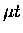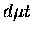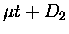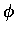Next: Subthreshold resonances Up: The standard Vesman model Previous: Decay of the molecular

### Total and effective formation rates

For our analysis, it is convenient to express the formation rates as a function of thelaboratory (lab) energy, as opposed to the target temperature given in Eq.  2.27. Specifying the initial and final states, we then have:(47)

where=(48)=(49)

whereis the initial rotational population distribution, which for equilibrated targets is the standard Boltzmann distribution, and Aif is a coefficient which depends on initial and final quantum numbers. The factoris the Doppler broadening profile due to target motion and recoil, whose exact form is derived in Ref. , but forcan be approximated  as a Gaussian distribution with the width of:(50)

In Eq. 2.35, afunction resonance profile was assumed (the classical Vesman model), but even with that, the formation rate in the lab frame has a distribution with non-zero width due to the above-mentioned Doppler broadening.

At epithermal energies, the transition formation matrix elements become very large, which means both the formation rate and back decay width are large, resulting in a significant probability fornot fusing but returning to the entrance channel. The effective formation rate is a renormalized rate taking into account the fusion probability, as defined in Ref. :(51)

where=(52)=(53)

For a high density (more than about 0.1) target such as ours, Faifman assumes complete rotational relaxation of the Kf levels, hence dropping the Kf dependence,=(54)=(55)=(56)

whereis the Boltzmann distribution of the Kf states.

The effective fusion probability for the molecular complex is defined as :(57)

whereis the rate for non-resonant formation, which does not back-decay. For, WF can be written:(58)

which can be understood as the average of fusion probabilities from each state weighted by the formation rate of that state.

Figure 2.4 shows the molecular formation ratesand effective fusion probabilities WF for Ki=0(ortho) and Ki=1 (para) cases, calculated by Faifman et al. [70,71,72] for a 3 K target. Also shown are the effective rates. Note thatless than about 107s-1 is invisible in the scale plotted.Next: Subthreshold resonances Up: The standard Vesman model Previous: Decay of the molecular